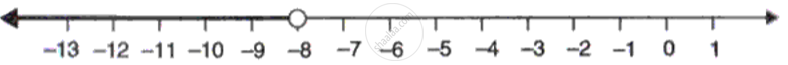Advertisement Remove all ads

# Solve the Following Linear In-equation and Graph the Solution Set on a Real Number Line: - Mathematics

Sum

Solve the following linear in-equation and graph the solution set on a real number line:

5/4 "x" > 1 + 1/3 (4"x" - 1) , x ∈ R

Advertisement Remove all ads

#### Solution

5/4 "x" > 1 + 1/3 (4"x" - 1)

5/4 "x" > (3 + (4"x" - 1))/3

15x > 12 + 16x - 4

15x - 16x > 8

- x > 8

x < -8

Solution set = [x < -8]Concept: Representation of Solution on the Number Line
Is there an error in this question or solution?
Advertisement Remove all ads

#### APPEARS IN

Frank ICSE Class 10 Mathematics Part 2
Chapter 5 Linear Inequations
Exercise 5.1 | Q 13.1 | Page 75
Advertisement Remove all ads
Advertisement Remove all ads
Share
Notifications

View all notifications

Forgot password?
Course